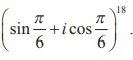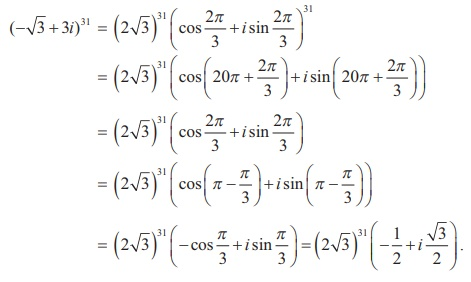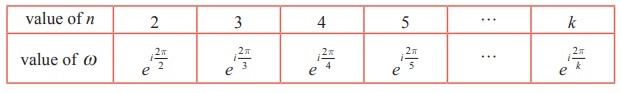Home | | Maths 12th Std | Solved Example Problems on de Moivre‚Äôs Theorem

# Solved Example Problems on de Moivre‚Äôs Theorem

Mathematics : Complex Numbers: Solved Example Problems on de Moivre‚Äôs Theorem

Example 2.28

If z = (cosŒ∏ + i sinŒ∏ ) , show that zn + 1/ zn = 2 cos nŒ∏ and zn ‚Äì [1/ zn] = 2i sin nŒ∏ .

Solution

Let z = (cosŒ∏ + i sinŒ∏ ) .

By de Moivre‚Äôs theorem ,

zn = (cosŒ∏ + i sinŒ∏ )n = cos nŒ∏ + i sin nŒ∏Example 2.29

Similarly,SolutionExample 2.30SolutionExample 2.31

Simplify

(i) (1+ i)18

(ii) (-‚àö3 + 3i)31 .

Solution

(i) (1+ i)18

Let 1+ i = (cosŒ∏ + sinŒ∏ ) . Then, we get(ii) (-‚àö3 + 3i)31 .

Let -‚àö3 + 3i = r (cosŒ∏ + i sinŒ∏ ) . Then, we getRaising power 31 on both sides,## The nth  roots of unity

Example 2.32

Find the cube roots of unity.

Solution

We have to find 11/3 . Let z = 11/3 then z3 = 1.

In polar form, the equation z3 = 1 can be written as

z3 = cos(0 + 2kœÄ) + i sin(0 + 2kœÄ) = ei2kœÄ , k = 0, 1, 2,...Example 2.33

Find the fourth roots of unity.

Solution

We have to find 11/4. Let z =11/4 . Then z4 = 1 .

In polar form, the equation z4 = 1 can be written as

z4 = cos (0 + 2kœÄ ) + i sin (0 + 2kœÄ ) = ei2kœÄ , k = 0, 1, 2,...Note

(i) In this chapter the letter œâ is used for nth roots of unity. Therefore the value of œâ is depending on n as shown in following table.(ii) The complex number zeiŒ∏ is a rotation of z by Œ∏ radians in the counter clockwise direction about the origin.

Example 2.34

Solve the equation z3 + 8i = 0 , where z ‚àà C

### Solution

Let z3 + 8i = 0 . Then, we get

z3 = -8i### Example 2.35

Find all cube roots of ‚àö3 +

Solution

We have to find (‚àö3 + i)1/3. Let z3 = ‚àö3 + i = r (cosŒ∏ + i sinŒ∏ )### Example 2.36

Suppose z1 , z2, and z3 are the vertices of an equilateral triangle inscribed in the circle |z| = 2. If z1 = 1+ i‚àö3 , then find z2 and z3.

### Solution

|z| = 2 represents the circle with centre (0, 0) and radius 2.

Let A, B, and C be the vertices of the given triangle. Since the vertices z1 , z2 , and z3 form an equilateral triangle inscribed in the circle |z| = 2 , the sides of this triangle AB, BC, and CA subtend 2œÄ/3 radians (120 degree) at the origin (circumcenter of the triangle).

(The complex number z eiŒ∏ is a rotation of z by Œ∏ radians in the counter clockwise direction about the origin.)

Therefore, we can obtain z2 and z3 by the rotation of z1 by 2œÄ/3 and 4 œÄ/3 respectively.

Given thatTherefore, z2 = -2, and z3 = 1- i‚àö3.

Study Material, Lecturing Notes, Assignment, Reference, Wiki description explanation, brief detail
12th Mathematics : UNIT 2 : Complex Numbers : Solved Example Problems on de Moivre‚Äôs Theorem |